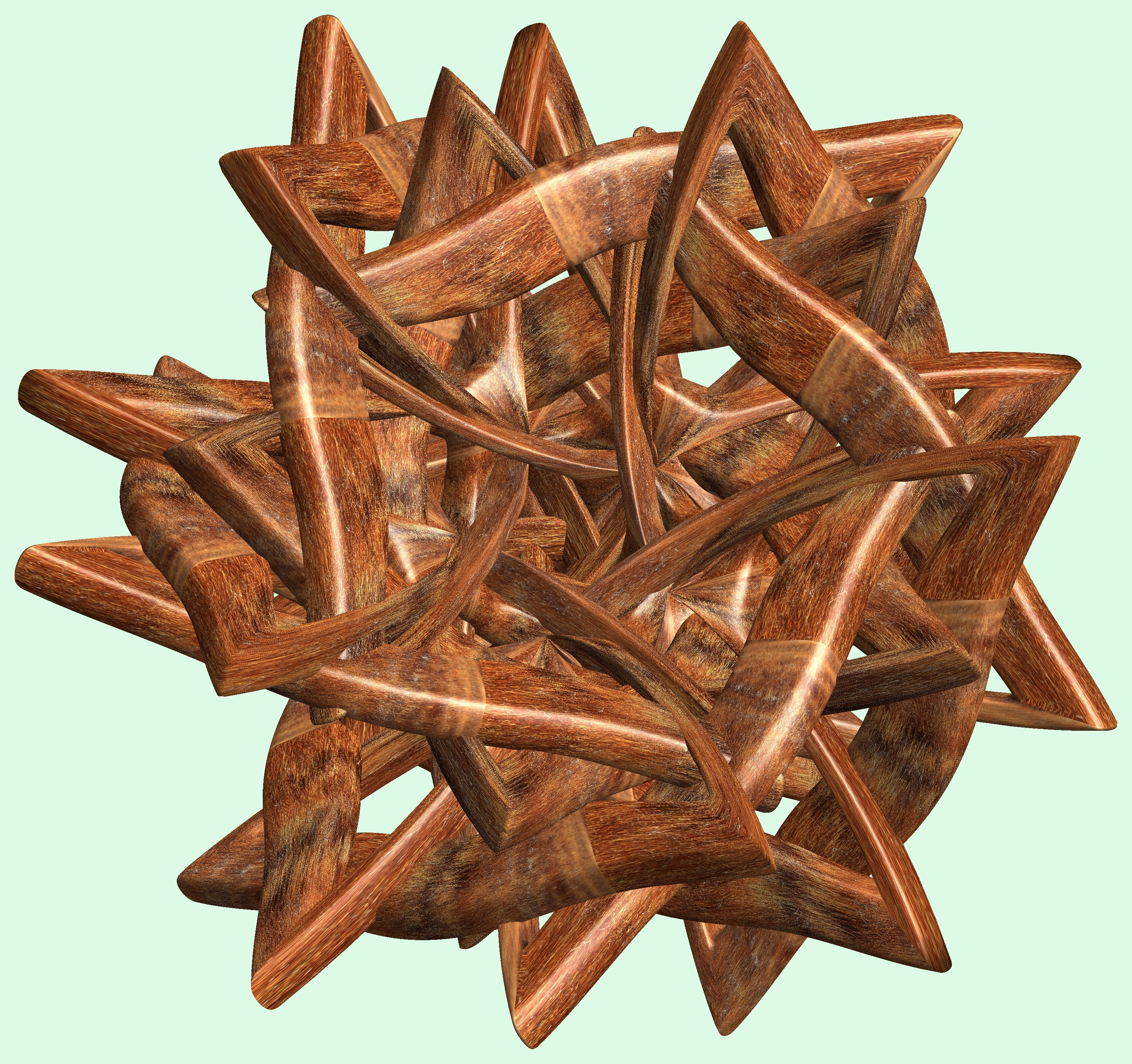# 5 Knots / ５つの結び目

###### Related for 5 Knots / ５つの結び目5 knots / ５つの結び目 free stock photo

(* === Following code of Mathematica 8 generates this image. === *)

a1 = 16; (* center hole size *)

a2 = 2; (* thickness of a torus *)

a3 = 5; (* wave height of a torus *)

b1 = 5;(*number of cross*)

b2 = 3;(*number of cross*)

c = 4; (* distance from the center of rotation *)

d = 5; (* number of torus *)

h = 1; (* height of a torus *)

SetOptions[ParametricPlot3D, PlotRange -> Full, Mesh -> None, Boxed -> False, Axes -> None,

PlotPoints -> 600, ImageSize -> 3000, Background -> RGBColor[{220, 250, 230}/255],

PlotStyle -> Directive[Specularity[White, 30], Texture[Import["D:/tmp/31.jpg"]]],

TextureCoordinateFunction -> ({#4, #5 b1 Pi} &), Lighting -> "Neutral"];

f[v_] := Sin[2 Sin[Sin[Sin[v]]]];

g[v_] := If[Sin[v] > 0, -3, 1];

x = (a1 - a2 Cos[t] + a3 g[b1 s] f[b1 s]) Cos[b2 s] + c;

y = (a1 - a2 Cos[t] + a3 g[b1 s] f[b1 s]) Sin[b2 s] + c;

z = h (Cos[b1 s] + f[t]);

rm = Table[{x, y, z}.RotationMatrix[2 i Pi/d, {0, 1, 1}], {i, d}];

ParametricPlot3D[rm, {t, 0, 2 Pi}, {s, 0, 2 Pi}]

(*---

The Texture

Another shapes and colors, and the meaning of this code in the album description

*)

Free high resolution images 5 knots / ５つの結び目, 3d, テクスチャ, たいしょう, たいしょうせい, ちゅうしょう.
Licence: Creative Commons - CC0. Photos transferred to the public domain from Creative Commons.

Comments:

## Related premium images308
0

### Flickr (Public Domain)

9174 images